# GSEB Solutions Class 12 Physics Chapter 15 Communication Systems

Gujarat Board GSEB Textbook Solutions Class 12 Physics Chapter 15 Communication Systems Textbook Questions and Answers, Additional Important Questions, Notes Pdf.

## Gujarat Board Textbook Solutions Class 12 Physics Chapter 15 Communication Systems

### GSEB Class 12 Physics Communication Systems Text Book Questions and Answers

Question 1.
Which of the following frequencies will be suitable for beyond the horizon communication using sky waves?
(a) 10 kHz
(b) 10 MHz
(c) 1 GHz
(d) 1000 Hz
(b) 10 kHz cannot be radiated (antenna size), 1 GHz and 1000 GHz will penetrate.

Question 2.
Frequencies in the UHF range normally propagate by means of:
(a) Ground waves.
(b) Sky waves.
(c) Surface waves.
(d) Space waves.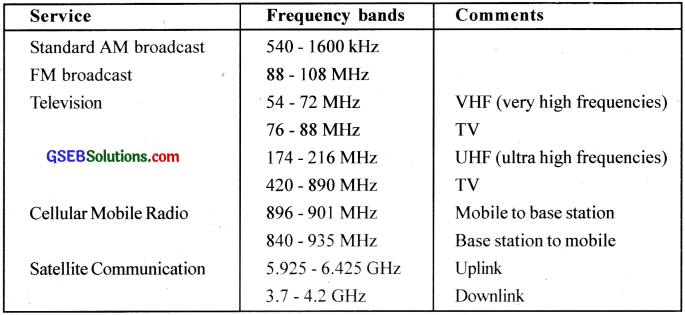Question 3.
Digital signals
i. do not provide a continuous set of values
ii. represent values as discrete steps
iii. can utilize binary system
iv. can utilize decimal as well as binary systems
Which of the above statements are true?
(a) (i) and (ii) only
(b) (ii) and (iii) only
(c) (i), (ii) and (iii) but not (iv)
(d) All of (i). (ii), (iii) and (iv).
(c) Decimal system implies continuous set of valuesQuestion 4.
Is it necessary for a transmitting antenna to be at the same height as that of the receiving antenna for line-of-sight communication? A TV transmitting antenna is 81 m tall. How much service area can it cover if the receiving antenna is at the ground level?
No. Service area will be A = π$${ d }_{ T }^{ 2 }$$ = $$\frac {22}{7}$$ x 162 x 6.4 x 106 = 3258 km2

Question 5.
A carrier wave of peak voltage 12V is used to transmit a message signal. What should be the peak voltage of the modulating signal in order to have a modulation index of 75%?
µ = 0.75 = $$\frac{A_{m}}{A_{c}}$$
Am = 0.75 x 12 = 9V

Question 6.
A modulating signal is a square wave, as shown in the figure.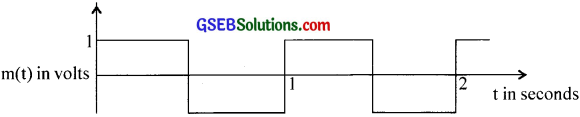The carrier wave is given by c(t) = 2sin(8πt) volts.
i. Sketch the amplitude modulated waveform
ii. What is the modulation index?
(a)(b) μ = 0.5

Question 7.
For an amplitude modulated wave, the maximum amplitude is found to be 10V while the minimum amplitude is found to be 2V. Determine the modulation index, t. What rould be the value of j-t ¡f the minimum amplitude is zero volt?
Since the AM wave is given by (Ac + Am sinωmt) cos ωct, the maximum amplitude is
M1 = Ac + Am while the minimum amplitude is M2 = Ac – Am Hence the modulation index is
m = $$\frac{A_{m}}{A_{c}}=\frac{M_{1}-M_{2}}{M_{1}+M_{2}}=\frac{8}{12}=\frac{2}{3}$$ With M2 = 0,clearly.m = 1, irrespective of M1.Question 8.
Due to economic reasons, only the upper side band of an AM wave ¡s transmitted, but at the receiving station, there is a facility for generating the carrier. Show that if a device is available which can multiply two signals, then it is possible to recover the modulating signal at the receiver station.
Let, for simplicity, the received signal be A1 cos(ωc + ωm )t
The carrier Ac cos ωct is available at the receiving station. By multiplying the two signals,
we get A1Ac cos(ωc + ωa)t cos ωct = $$\frac{\mathrm{A}_{1} \mathrm{~A}_{\mathrm{c}}}{2}$$ [cos(2ωc + ωm)t + cosωmt]
If this signal is passed through a low-pass filter, we can record the modulating signal = $$\frac{\mathrm{A}_{1} \mathrm{~A}_{\mathrm{c}}}{2}$$ cosωmt

### GSEB Class 12 Physics Communication Systems Additional Important Questions and Answers

Question 1.
flow will you classifi.’ communication systems?
Communication systems can be classified based on the nature of source. mode of communication. type of modulation and nature of channel used.

Question 2.
What are the lips of channels used for transmission?
i. Space communication (Broadcasting, microwave mobile etc.)
ii. Line communication (Two wire, co-axial cables, fiber optical etc.)

Question 3.
What is the length of antenna required to transmit wave of frequency 40 Hz and 40 MHz?
The minimum length of antenna required is $$\frac {λ}{4}$$
Velocity,c = υλ
λ = $$\frac {c}{υ}$$Question 4.
Identify the sound that can travel a longer distance – siren from a factory or horn of a car. Why?
Siren from a factory. High intensity.

Question 5.
Mention the factors on which power of electromagnetic wave transmitted depends.
The power of electromagnetic wave is related to the length of antenna and wavelength of the wave.
Power α $$\left(\frac{l}{\lambda}\right)^{2}$$, where l is the length and λ-the wavelength.

Question 6.
Which range of wave is more reliable of intermixing – shorter or longer wavelength?
Longer wavelengthQuestion 7.
is there any change in the frequency or phase due to amplitude modulation?
No change of either frequency or phase.

Question 8.
Which physical quantity of wave is varied in AM. FM and PM?
In AM. the physical quantity of carrier that changes is amplitude.
In FM, the physical quantity of carrier that change is frequency.
In PM, the physical quantity of carrier that changes is phase.

Question 9.
What are the advantages and limitations of AM and FM?

 Advantages Limitations AM Wireless transmission possible, simple circuit, two sided bands Low efficiency, small operating range. noisy receptions, interference effect. FM More resistant to noise, large number of side bands, carrier frequency high (television broadcast), more economical space wave propagation Bandwidth wide, circuit more complex, smaller area of reception

Question 10.
What is ground wave propagation?
Ground wave follows curvature of the earth and has carrier frequencies up to 2MHz. e.g. AM radio.
Ground waves progress along the surface of the earth and must be vertically polarized to prevent short circuiting the electric equipments. A wave induces currents in the ground over which it passes and thus loses some energy by absorption. This is made up by energy diffracted downwards from the upper portion of the wave front.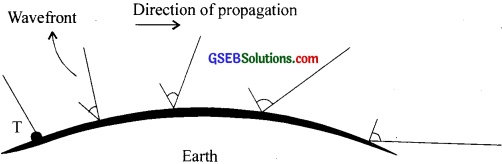There is another way also by which the ground waves get attenuated. Because of diffraction, the wave front gradually tilts over, as shown in figure. As the wave propagates over the earth, the tilt increases and this tilt causes greater short-circuiting of the electric component of the wave. Hence there is a reduction in the field strength. Eventually at some distance from the antenna, the wave gets weakened and dies off. The maximum range of such a transmitter depends on its frequency and power. The ground wave propagation is effective only at VLF.

Question 11.
Ground waves are not sustained for a long range communication. Why?
Because of damping by earth surface.

Question 12.
What is the range of frequencies used in ground wave propagation? Why?
VLF. The attenuation of surface waves increases very rapidly with increase in frequency.

Question 13.
How can we overcome this limitation?
By changing to space wave communication.

Question 14.
What is the basic requirement of space wave communication?
There should be transmitter and receiving antenna.

Question 15.
Why is space communication also known as line of sight communication?
The transmitting and receiving antenna are on sight.Question 16.
Why are repeaters needed in line of sight communication?
To compensate for loss of energy during propagation.

Question 17.
What are the limitations of space wave propagation?
This method needs repeaters and suitable antenna length (height).

Question 18.
What is sky wave communication?
Sky wave communication is otherwise called ionospheric communication. In this the electromagnetic wave of high frequency is directed towards the ionosphere which reflects the wave back to earth.

Question 19.
Which space transmission technology makes use of total internal reflection?
ionospheric transmission.

Question 20.
is sky wave propagation possible on moon? Why?
No. Moon has no ionosphere.

Question 21.
Can all frequencies be transmitted using sky wave propagation?
No. Only frequencies below the critical value.

Question 22.
How does sky wave propagation depend on refractive index of atmosphere?
The refractive index of ionosphere decreases below that of free space by the change of velocity of electrons in the ionosphere and the electromagnetic saves undergo total internal reflection.

Question 23.
Through which atmospheric layer. does the propagation take place in ground. space and sky communications?
Ground wave – Troposphere
Space wave – Troposphere
Sky wave – Ionosphere

Question 24.
Which type of space communication has maximum range of transmission?
SatelliteQuestion 25.
Compare the principle applied for each type of communication.
Ground wave – Wireless
Space wave – Line of sight
Sky wave – Total internet reflection by ionosphere.

Question 26.
What is the range of frequency used ¡n each case?
Ground wave – <2 MHz
Space wave -> 30 MHz
Sky wave -< 10 MHz

Question 27.
Point out the limitations and uses in each case.
Ground wave – Damping effect, wireless communication.
Space wave – Finite curvature of earth, line of sight
Sky wave – Critical frequency, long distance coverage

Question 28.
Name the type of channel used in telephone, cable TV and high speed internet connections.
Telephone – Two wire
Cable TV – Coaxial cable
Internet – Space (satellite) .

Question 29.
Which ¡s the cheapest mode of line communication?
Two wire system

Question 30.
What are the merits and demerits of two wire communication?
Signals can travel kilometres without amplification, digital and analogue signals can be sent cheap.
Attenuation of signal, interference etc.

Question 31.
Why twisted wires are preferred?
To reduce interference of electromagnetic radiations.Question 32.
Under which condition, does maximum power transmission occur through two wire lines?
When the impedance of the detecting device at the receiver (load) is matched (i.e., equal) to the characteristic impedance of the two wire system.

Question 33.
What kind of cable is used to connect VC’R to TV?
Coaxial

Question 34.
Draw the figure of coaxial cable.Question 35.
What is the structure of coaxial cable?
Coaxial cables are shielded i.e., outer conductor surrounds the insulated inner wire and the outer is always grounded.

Question 36.
Which type of material  is suitable to use as spacer in coaxial cable? Why?
Solid dielectric material, for insulation.

Question 37.
What are the merits of coaxial cables?
These cables do not suffer from radiation problem and can he used for microwaves.

Question 38.
For establishing a communication between a transmitting and receiving station, a physical medium is used.
(a) Name the two principal classes of communication based on the physical medium used for propagation.
(b) Construct a table showing advantages and one practical application each for the two wire, coaxial cable and optic fiber communication.
(c) in cable TV transmission usually channel in UHF band carries relatively more noise, compared to VHF band. Justify
(a) Line communication and space communication.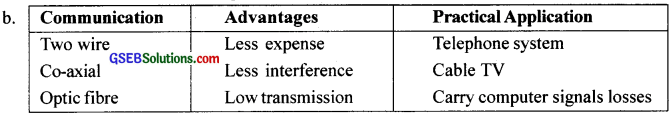(c) At higher frequency, radiation loss is high.

Question 39.
Schematic diagram for three types of satellite orbits are shown below and named as A.B.C.(a) Identify the polar orbit and give its approximate height from earth.
(b) Give the criteria for selecting frequency of em wave to be used in photographs from satellites.
(c) A satellite T V company attempts to use 25,000 kHz for up linking signal to a sat ellite. Say whether they have selected apt frequency. Justify.
(a) Orbit C. Its height is about 1000 km.
(b) i. Nature of the atmosphere.
ii. Reluctance of the object.
(c) No. Because frequency below 20 MHz will undergo total internal reflection at the ionosphere.Question 40.
The following diagrams represent some of ihe modulated signals.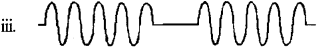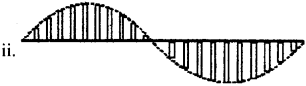Which among is following correct
a. i only
b. ii only
c. iii only
d. both i and ii
d. both i and ii

Question 41.
in communication the concept of information is the central thing. Instead of information we deal with messages, since there is no precise definition for the word information.
(a) Name the two distinct message categories.
(b) Explain them with examples.
(a) In electronic communication systems, we use
i. analog
ii. digital signals

(b) i. Analog signal is continuous in amplitude and time variables. e.g. Speech converted microphone signal, the ECO etc.
ii Digital – This signal is discrete in amplitude and time. Here, the analog signal is subjected to time-sampling and amplitude quantization. e.g. Digital video stream, Data files etc.

Question 42.
List the various types of communications according to
(a) nature of information
(b) mode of transmission
(c) transmission channel
(d) types of modulation
(a) Speech. picture, fax, data transmission
(b) Analog and digital communication
(c) i. Space communication
ii. Line communication
(d) i. Sinusoidal waves – AM, FM, PM
ii. Pulsed carrier waves – PAM, PTM, PPM, PNM, PCM

Question 43.
Explain the necessity of modulation.
The unmodified signal from source will be usually weak to be transmitted to long distance through channel. The Long-wave signal is then suitably combined with a high frequency (short wave) wave called carrier. During combining some property of the carrier is allowed to vary in proportion to that of the signal. This process is called modulation.Question 44.
(a) What is meant by demodulation?
(b) What is its necessity?
(c) What are the different types of demodulation?
(a) The process of extracting the information from a modulated wave is called demodulation or detection.
(b) Demodulation is an essential process for realization at the receiving end.
(c) There are different types of detectors depending on the type of modulation. e.g. optical detector, diode detector etc.

Question 45.
(a) What does the figure represent?
(b) What is the function of ‘C’?
(c) What is the function of ‘R’?(a) This is an AM demodulate (or detector)
(b) The capacitor charges to the peak voltage and then discharges through R. It serves as a capacitor filter. The diode rectifies the incoming AM signal. The rectified wave, while passing through the network, gets the RF carrier component removed thus producing the original signal.
(c) The voltage across R is the envelope of modulated wave (the signal)

Question 46.
Space wave communication is called troposphere wave propagation or LOS.
It takes place at line of sight condition.

Question 47.
Name the four areas in which space technology finds application.
Meteorology, climatology, oceanography and coastal studies

Question 48.
“For long TV transmission we need satellites “. Give reason.
The bandwidth of picture from TV camera is about 64 Hz. Because of various factors that alternate the wave, a signal directly transmitted may not reach the destination.

Question 49.
Flow Kepler’s III law plays an important role in satellite communication?